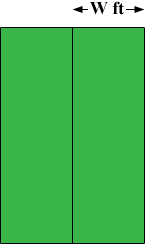SEARCH HOMEMath Central Quandaries & QueriesQuestion from Janet, a student: Clint is constructing two adjacent rectangular dog pens. Each pen will be three times as long as it is wide, and the pens will share a common long side. If Clint has 65 ft of fencing, what are the dimensions of each pen?Hi Janet,

Draw a diagram and label the width of one of the pens as W feet.What is the length of the pens? Label all the sides. What is the total lengths of the sides? This total is 65 feet. Solve for W.

PennyMath Central is supported by the University of Regina and The Pacific Institute for the Mathematical Sciences.